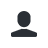# Math 1.0 v4+

1.Math
Why?
Why wouldn't you want an advanced Mathematics engine ready at short notice? So many possible uses, and no performance impact. You never know when you might need to perform a quick calculation. Math will be there.How powerful is it?
Really powerful. It can handle variables, complex expressions, and so much more because it is based on the exp4j Mathematics engine. Math can handle expressions as simple as 5+5, all the way to (2cos(x*y)%sin(x))*sqrt(32),x=43,y=44. You can even store and handle global variables. Store server variables that anyone can access. Store expressions as variables that you can reuse. Math can do it.

How do I use it?
It's easy. Just use /exp <expression>,[variables...]. For example, /exp 5+5. Or, /exp x+y/x,x=5,y=2. Or /exp log(2cos(x*y)%sin(x))*sqrt(32),x=43,y=44. Or, if you wanted to write a global variable, use /var def x=5. Now x can be used in expressions. Or you could store the value of an expression with /exp 5+5 ->x. If you wanted to define a server variable, capitalize the variable name. Others will be able to use, but not access, the variable.

In a nutshell, you can use /exp <expression>. If you're using variables, use /exp <expression>,<variables defined like x=5,y=3> [->(variable)]

Need to be quick? Use /math auto. Now, everything you say in chat will be read as an expression to evaluate. Disable by saying 'exit' or running /math auto again.

There is only one permission for this plugin: math.use

If you want to be able to store variables and reuse them, use /var def <variables...>, where <variables...> are defined normally. For example, /var def x=5 would make /exp x+1 evaluate to 6. It's important that you can override global variables by redefining them in the expression (/exp x+1,x=7 would return 8 even though x is globally set to 5). Additionally, variable names that are capitalized can be accessed by anyone.

Detailed usage
/exp:
Code (Text):

[18:21:56 INFO]: [MATH] Help ---------------------------------------

[18:21:56 INFO]: [MATH] Command usages:

[18:21:56 INFO]: [MATH]     /math var <def/my/clear>|exp <expression> [variables...]|auto // gateway command

[18:21:56 INFO]: [MATH]     /exp <expression> [variables...] // evaluate expressions

[18:21:56 INFO]: [MATH]       /exp 5+5 // evaluate 5+5

[18:21:56 INFO]: [MATH]       /exp x/y,x=5,y=6 // evaluate x/y where x=5, y=6

[18:21:56 INFO]: [MATH]     /var def|my|clear // define global variables

[18:21:56 INFO]: [MATH] Example simple expressions:

[18:21:56 INFO]: [MATH]     5 + 3

[18:21:56 INFO]: [MATH]     3 * sin(5) - 2 / (1 - 2)

[18:21:56 INFO]: [MATH] Example variable expressions:

[18:21:56 INFO]: [MATH]     x+5,x=1

[18:21:56 INFO]: [MATH]     5 * sin(x) - (3 - z),x=5,z=4

[18:21:56 INFO]: [MATH]     (declare variables in the form of <variable>=<value>,...)

[18:21:56 INFO]: [MATH] Built-in functions:

[18:21:56 INFO]: [MATH]     view /exp functions

[18:21:56 INFO]: [MATH] Want even more? Read library documentation @ http://projects.congrace.de/exp4j/

available functions:
Code (Text):
[16:05:15 INFO]: [MATH]     - abs: absolute value
[16:05:15 INFO]: [MATH]     - acos: arc cosine
[16:05:15 INFO]: [MATH]     - asin: arc sine
[16:05:15 INFO]: [MATH]     - atan: arc tangent
[16:05:15 INFO]: [MATH]     - cbrt: cubic root
[16:05:15 INFO]: [MATH]     - ceil: nearest upper integer
[16:05:15 INFO]: [MATH]     - cos: cosine
[16:05:15 INFO]: [MATH]     - cosh: hyperbolic cosine
[16:05:15 INFO]: [MATH]     - exp: euler's number raised to the power (e^x)
[16:05:15 INFO]: [MATH]     - floor: nearest lower integer
[16:05:15 INFO]: [MATH]     - log: logarithmus naturalis (base e)
[16:05:15 INFO]: [MATH]     - log10: logarithm (base 10)
[16:05:15 INFO]: [MATH]     - log2: logarithm (base 2)
[16:05:15 INFO]: [MATH]     - sin: sine
[16:05:15 INFO]: [MATH]     - sinh: hyperbolic sine
[16:05:15 INFO]: [MATH]     - sqrt: square root
[16:05:15 INFO]: [MATH]     - tan: tangent
[16:05:15 INFO]: [MATH]     - tanh: hyperbolic tangent
/var:
Code (Text):
[16:06:13 INFO]: [MATH] Define variables like /var def x=5
[16:06:13 INFO]: [MATH] Clear all with /var clear
[16:06:13 INFO]: [MATH] Remove individual variables with /var rem <variable>
[16:06:13 INFO]: [MATH] View your variables with /var my
ImDaFatBoss and ferrinweb like this.

1. SERVER VARIABLES!

### Recent Reviews

1.Pawelgo
5/5,
Version: 1.0 v4+
Great! Still works in 1.17, after 7 years. And it's the best one on spigotmc. No need to pull out my casio to figure out how long a ban should be now.
2.Mikioul
5/5,
Version: 1.0 v4+
Thanks You so much ! Very nice plugin .
3.ASCIIcat
5/5,
Version: 1.0 v3
This is actually exactly what I have been looking for. There have been so many times I have needed to work something out while on the server, but been too lazy to look for my calculator!
1.Author's Response
4.